## Deutsch-Jozsa Algorithm and the Hadamard Sandwich - Quantum Computer Programming

Quanum-prog-3-deutsch-jozsa-FINAL

Greetings and welcome to the 3rd Quantum Computer programming tutorial. At this point, hopefully you have a decent grasp of what a qubit is, what gates are, and of the magnitude of possibilities here. You should also have some idea and feeling of how the overall computing paradigm is much different when comparing quantum computers and classical computers.

I would now like to introduce you to one of the characteristics of Quantum computing that is the basis and inspiration for many algorithms. In order to do this though, I would like to show you why it's the basis and/or inspiration. When I came across this algorithm, I saw countless examples and walkthroughs, but no one actually explained and showed in depth why and how this was impressive. So first, I will outline the problem, then we'll get cracking away at it!

The premise goes something like this:

Given some black box, f(), how many passes through the circuit will it take to know if this black box is balanced (returns even # of 1s and 0s given input) or constant (returns all 1s or all 0s always)?

It depends on the number of bits we pass, so let's assume we're using a 2-bit string for now.

This is a task that would take a classical computer at least 2 tries to figure out. The more bits that you have, however, the more tries it will take before the classical machine can be confident.

A Quantum computer, however, can do this in 1 shot. No matter how many bits we're passing through this function.

This is the Deutsch-Jozsa Algorithm.

Let's start off with some necessary imports:

import qiskit as q
from qiskit.visualization import plot_histogram, plot_bloch_multivector
from matplotlib import style
import math
#style.use("dark_background")
%matplotlib inline

qasm_sim = q.Aer.get_backend('qasm_simulator')
statevec_sim = q.Aer.get_backend("statevector_simulator")


Now, before we get into our black box situation, I would first like to talk about sandwiches.

Very interesting things happen when we "sandwich" our quantum circuits in Hadamard Gates...and yes this will be useful to our problem.

Let's define a simple circuit:

### Original Circuit of uncertain qubits:¶

c = q.QuantumCircuit(2,2)
c.ry(math.pi/4,0)
c.ry(math.pi/4,1)
orig_statevec = q.execute(c, backend=statevec_sim).result().get_statevector()
c.measure([0,1], [0,1]) # measuring qubit 3, which is impacted by those cnots:
c.draw()

        +----------++-+
q_0: |0>+ Ry(pi/4) ++M+---
+----------+++++-+
q_1: |0>+ Ry(pi/4) +-+-+M+
+----------+ | +++
c_0: 0 -------------+--+-
|
c_1: 0 ----------------+-

plot_bloch_multivector(orig_statevec)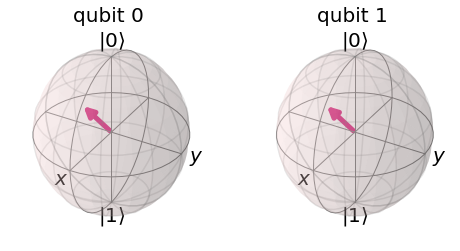What's this output for counts?

orig_counts = q.execute(c, backend=qasm_sim, shots=1024).result().get_counts()
plot_histogram([orig_counts], legend=['output'])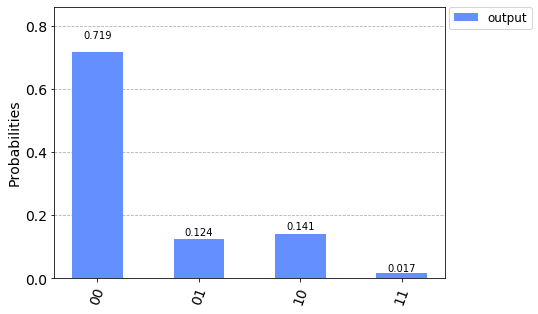Take note of both the multivector and the histogram. We've saved both as orig_ versions so we can reference them later.

### Hadamards in front of uncertain qubits:¶

c = q.QuantumCircuit(2,2)
c.h(0)
c.h(1)
c.ry(math.pi/4,0)
c.ry(math.pi/4,1)
statevec = q.execute(c, backend=statevec_sim).result().get_statevector()
c.measure([0,1], [0,1]) # measuring qubit 3, which is impacted by those cnots:
c.draw()

        +---++----------++-+
q_0: |0>+ H ++ Ry(pi/4) ++M+---
+---++----------+++++-+
q_1: |0>+ H ++ Ry(pi/4) +-+-+M+
+---++----------+ | +++
c_0: 0 ------------------+--+-
|
c_1: 0 ---------------------+-

plot_bloch_multivector(statevec)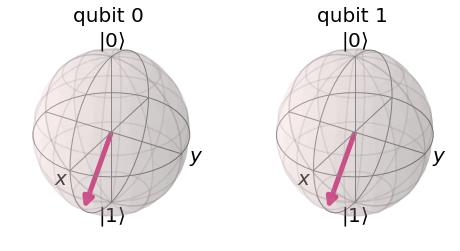counts = q.execute(c, backend=qasm_sim, shots=1024).result().get_counts()
plot_histogram([counts], legend=['output'])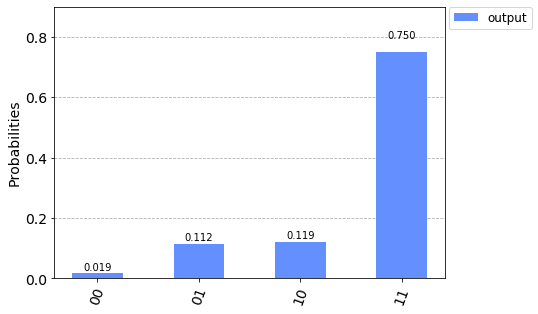This changes our distribution seemingly by reversing the probabilities for 00 and 11.

### Hadamard Sandwich of uncertain qubits!¶

c = q.QuantumCircuit(2,2)
c.h(0)
c.h(1)
c.ry(math.pi/4,0)
c.ry(math.pi/4,1)
c.h(0)
c.h(1)
statevec = q.execute(c, backend=statevec_sim).result().get_statevector()
c.measure([0,1], [0,1]) # measuring qubit 3, which is impacted by those cnots:
c.draw()

        +---++----------++---++-+
q_0: |0>+ H ++ Ry(pi/4) ++ H ++M+---
+---++----------++---+++++-+
q_1: |0>+ H ++ Ry(pi/4) ++ H +-+-+M+
+---++----------++---+ | +++
c_0: 0 -----------------------+--+-
|
c_1: 0 --------------------------+-


plot_bloch_multivector(statevec)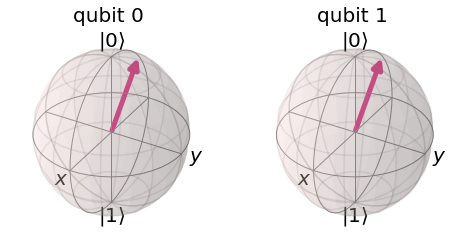Compared to the original:

plot_bloch_multivector(orig_statevec)These definitely look different.

...but how do they collapse?

counts = q.execute(c, backend=qasm_sim, shots=1024).result().get_counts()
plot_histogram([counts], legend=['output'])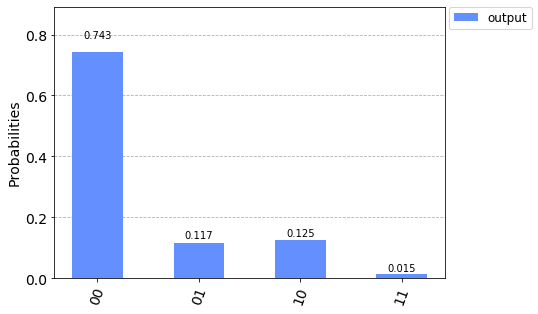plot_histogram([orig_counts], legend=['output'])Now... THAT is (or at least should be) interesting. This Hadamard sandwich appears to cause something to occur to the qubit, but not the distribution/output.

Note that we started with qubits and gates that were uncertain. That rotation on y gave us qubits that would collapse one way sometimes, and another way sometimes. What about qubits that are constants (either 1 or a 0)?

### Original circuit w/ certain qubits:¶

c = q.QuantumCircuit(2,2)
c.x(0)
c.x(1)
orig_statevec = q.execute(c, backend=statevec_sim).result().get_statevector()
c.measure([0,1], [0,1]) # measuring qubit 3, which is impacted by those cnots:
orig_counts = q.execute(c, backend=qasm_sim, shots=1024).result().get_counts()
plot_histogram([orig_counts], legend=['output'])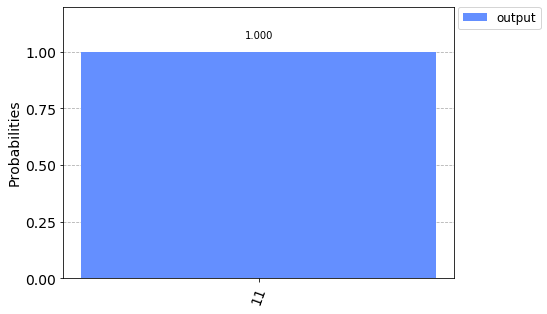Again, expected I think.

plot_bloch_multivector(orig_statevec)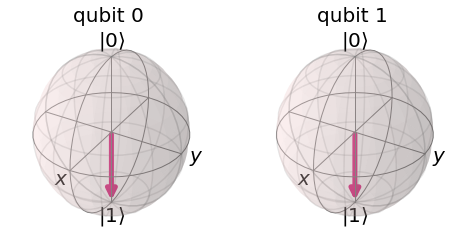### Hadamards in front of Certain Qubits:¶

c = q.QuantumCircuit(2,2)
c.h(0)
c.h(1)
c.x(0)
c.x(1)
statevec = q.execute(c, backend=statevec_sim).result().get_statevector()
c.measure([0,1], [0,1]) # measuring qubit 3, which is impacted by those cnots:
counts = q.execute(c, backend=qasm_sim, shots=1024).result().get_counts()
plot_histogram([counts], legend=['output'])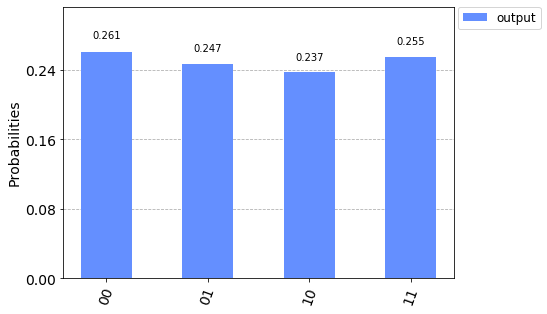I think this should be expected. A combination of every possibility.

... now, try to imagine what you think will happen with a full Hadamard sandwich...

### Hadamard sandwich of certain qubits:¶

c = q.QuantumCircuit(2,2)
c.h(0)
c.h(1)
c.x(0)
c.x(1)
c.h(0)
c.h(1)
statevec = q.execute(c, backend=statevec_sim).result().get_statevector()
c.measure([0,1], [0,1]) # measuring qubit 3, which is impacted by those cnots:
counts = q.execute(c, backend=qasm_sim, shots=1024).result().get_counts()
plot_histogram([counts], legend=['output'])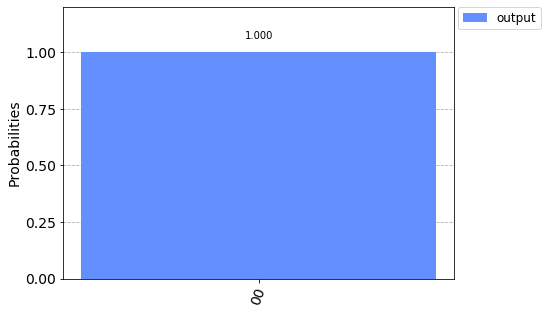Originally:

plot_histogram([orig_counts], legend=['output'])Despite the superpositions, all that seems to have happened is the output distribution went from 100% 00 to 100% 11, which is curious since the Hadamard Sandwich seemed to keep the same probability distribution when it came to uncertain qubits.

With this interesting nature of the Hadamard sandwich, we shall revisit our original problem of figuring out if a circuit is balanced or constant now.

For our constant "function" we can... just do nothing.

For our balanced function, we can use the controlled not/cnot/cx gate, entanged to some 3rd qubit. Let's setup our circuit and function "black boxes."

def balanced_black_box(c):
c.cx(0,2)
c.cx(1,2)
return c

def constant_black_box(c):
# outputs whatever you put in.
return c


Nothing special here, other than the balanced box is dependent on this entangled relationship with qubit at index 0, which will soon be in superposition.

c = q.QuantumCircuit(3,2)
c = balanced_black_box(c)
c.draw()


q_0: |0>--#-------
|
q_1: |0>--+----#--
+-+-++-+-+
q_2: |0>+ X ++ X +
+---++---+
c_0: 0 ----------

c_1: 0 ----------


c = q.QuantumCircuit(3,2)
c.h(0)
c.h(1)
c.h(2)
c = balanced_black_box(c)
c.h(0)
c.h(1)
# no need for ending H on q
c.draw()

        +---+     +---+
q_0: |0>+ H +--#--+ H +-----
+---+  |  +---++---+
q_1: |0>+ H +--+----#--+ H +
+---++-+-++-+-++---+
q_2: |0>+ H ++ X ++ X +-----
+---++---++---+
c_0: 0 --------------------

c_1: 0 --------------------


Things are starting to look messy, so let's use barrier for clarity:

c = q.QuantumCircuit(3,2)
c.h(0)
c.h(1)
c.h(2)
c.barrier()
c = balanced_black_box(c)
c.barrier()
c.h(0)
c.h(1)
# no need for ending H on q
c.draw()

        +---+ #            # +---+
q_0: |0>+ H +-#---#--------#-+ H +
+---+ #   |        # +---+
q_1: |0>+ H +-#---+----#---#-+ H +
+---+ # +-+-++-+-+ # +---+
q_2: |0>+ H +-#-+ X ++ X +-#------
+---+ # +---++---+ #
c_0: 0 --------------------------

c_1: 0 --------------------------


The barrier is meant to be for having some control over the backend optimizer for quantum circuits before they go to the actual quantum machines, but they have the side-benefit of helping us to visualize drawn circuits cleanly.

Now we just need to add the finishing touch. We want to apply a not before our qubit at index 2's hadamard. Before we do this, let's show what that does.

c = q.QuantumCircuit(1,1)
c.h(0)
statevec = q.execute(c, backend=statevec_sim).result().get_statevector()
plot_bloch_multivector(statevec)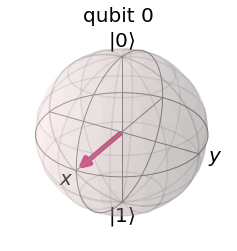### Not gate then a Hadamard:¶

c = q.QuantumCircuit(1,1)
c.x(0)
c.h(0)
statevec = q.execute(c, backend=statevec_sim).result().get_statevector()
plot_bloch_multivector(statevec)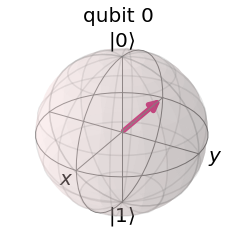So now you can see what's actually happening. It may not be what you expected ;).

Can you think of another way to achieve this exact same multivector?

I'll show you at the end. Try to think of it though.

Alright, back to our circuit, adding this not gate:

c = q.QuantumCircuit(3,2)
c.x(2)  # adding this not gate
c.barrier() # barrier for clarity
c.h(0)
c.h(1)
c.h(2)
c.barrier()
c = balanced_black_box(c)
c.barrier()
c.h(0)
c.h(1)
# no need for ending H on q... even though it'd make the sandwich look better :(
c.draw()

              # +---+ #            # +---+
q_0: |0>------#-+ H +-#---#--------#-+ H +
# +---+ #   |        # +---+
q_1: |0>------#-+ H +-#---+----#---#-+ H +
+---+ # +---+ # +-+-++-+-+ # +---+
q_2: |0>+ X +-#-+ H +-#-+ X ++ X +-#------
+---+ # +---+ # +---++---+ #
c_0: 0 ----------------------------------

c_1: 0 ----------------------------------


Now we just measure! Qubit is just there to get entangled with in that negative hadamard (?) state. I really do not know what to call it, but, visually, that's what I am going to go with.

c = q.QuantumCircuit(3,2)
c.x(2)  # adding this not gate
c.barrier() # barrier for clarity
c.h(0)
c.h(1)
c.h(2)
c.barrier()
c = balanced_black_box(c)
c.barrier()
c.h(0)
c.h(1)
c.measure([0,1],[0,1])
# no need for ending H on q... even though it'd make the sandwich look better :(
c.draw()

              # +---+ #            # +---++-+
q_0: |0>------#-+ H +-#---#--------#-+ H ++M+---
# +---+ #   |        # +---+++++-+
q_1: |0>------#-+ H +-#---+----#---#-+ H +-+-+M+
+---+ # +---+ # +-+-++-+-+ # +---+ | +++
q_2: |0>+ X +-#-+ H +-#-+ X ++ X +-#-------+--+-
+---+ # +---+ # +---++---+ #       |  |
c_0: 0 -----------------------------------+--+-
|
c_1: 0 --------------------------------------+-


Alright, that's it. Show time!

### Balanced Counts:¶

counts = q.execute(c, backend=qasm_sim, shots=1024).result().get_counts()
plot_histogram([counts], legend=['output'])And now for our constant box:

c = q.QuantumCircuit(3,2)
c.x(2)
c.barrier()
c.h(0)
c.h(1)
c.h(2)
c.barrier()
c = constant_black_box(c)  # changed to constant box.
c.barrier()
c.h(0)
c.h(1)
c.measure([0,1],[0,1])
c.draw()

              # +---+ #  # +---++-+
q_0: |0>------#-+ H +-#--#-+ H ++M+---
# +---+ #  # +---+++++-+
q_1: |0>------#-+ H +-#--#-+ H +-+-+M+
+---+ # +---+ #  # +---+ | +++
q_2: |0>+ X +-#-+ H +-#--#-------+--+-
+---+ # +---+ #  #       |  |
c_0: 0 -------------------------+--+-
|
c_1: 0 ----------------------------+-


### Constant Counts:¶

counts = q.execute(c, backend=qasm_sim, shots=1024).result().get_counts()
plot_histogram([counts], legend=['output'])### Okay... So what's this mean again?¶

The use of this Hadamard Sandwich (again this really is just a term I whipped up for this. It's not an official term and there's probably a better one that exists) has shown us one of the powers of Quantum Computing, where we can, intra-circuit, analyze other properties of the gates/circuit overall, all in one pass.

The actual problem of analyzing balanced/constant is pointless, as the actual value here is instead the showcasing and understanding of what this quantum circuitry can actually do, which is logically consider things while still in superposition and entanglement before the measurements collapse everything.

### Why does this matter?¶

Our method here gave us the opportunity to return a result that, instead of returning a function of input, it returned to us a function that described the nature of our circuit and data within it, in full, given any input.

That's bonkers.

Note that:

In the case of the balanced box, we got 11 for every single one of the 1024 shots. This means, in one shot, we could determine if a circuit was balanced or constant if the box is a balanced box.

In the case of a constant box, we got a 00 for every single one of the 1024 shots. This also means that, in one shot, we could determine if a circuit was balanced or constant in the case of a constant box too.

On a classical machine, determining this would take at least 2 passes, going up the more bits we add. In the case of a quantum computer, no matter how many input bits we had, we could figure this out in one pass.

Again, I am sure this alone is not too exciting to you, because the use-case is not immediately apparent.

... but it is useful. This is the first time that we can see a circuit, all in one shot, considering all of the possible combinations of that circuit, which is also us seeing the circuit itself being able to analyze attributes of itself.

This was the first Quantum algorithm, which was used to prove that quantum computers would be provably faster than a classical computer on at least this one task.

This algorithm influenced Shor's algorithm (the encryption breaking one) as well as the Bernstein-Vazirani algorithm (used to encode a number into a circuit).

In fact, you already basically know the Bernstein-Vazirani algorithm. You can encode any number to a circuit, because every controlled not gate, targetting the not+hadamard will output a 1 every time. Thus, you just do this for every 1 you want, and nothing for every 0, and this circuit will always output that.

FINALLY... I said I would bring back up this not+hadamard at the end. I asked you if there was another way. As a reminder:

c = q.QuantumCircuit(1,1)
c.x(0)
c.h(0)

statevec = q.execute(c, backend=statevec_sim).result().get_statevector()
plot_bloch_multivector(statevec)Recall that we've got: superpositions, entanglements, and rotations that make up all gates. So if we've got a qubit initialized to 0, and we want to get it to the same bloch vector as above, how would we do it? Well....

c = q.QuantumCircuit(1,1)
c.ry(math.pi,0)
c.h(0)

statevec = q.execute(c, backend=statevec_sim).result().get_statevector()
plot_bloch_multivector(statevec)Looks the same to me. Does it behave the same?

c = q.QuantumCircuit(3,2)

c.barrier()
c.ry(math.pi,2)  # changed c.x(2) to this.
c.h(0)
c.h(1)
c.h(2)
c.barrier()
c = balanced_black_box(c)
c.barrier()
c.h(0)
c.h(1)
c.measure([0,1],[0,1])

counts = q.execute(c, backend=qasm_sim, shots=1024).result().get_counts()
plot_histogram([counts], legend=['output'])And now:

c = q.QuantumCircuit(3,2)
c.ry(math.pi,2)  # changed c.x(2) to this.
c.barrier()
c.h(0)
c.h(1)
c.h(2)
c.barrier()
c = constant_black_box(c)
c.barrier()
c.h(0)
c.h(1)
c.measure([0,1],[0,1])

counts = q.execute(c, backend=qasm_sim, shots=1024).result().get_counts()
plot_histogram([counts], legend=['output'])Does this surprise you? Should it? Is a not gate merely the same as c.ry(math.pi,2)?

... well what if we move this gate?

I was curious because everywhere I looked had everyone using this not gate immediately, before the Hadamard for the extra qubit that gets entangled with all the others.

But I was interested to see if you could move the not gate to after the hadamard, and how this would impact things. Turns out, it does:

c = q.QuantumCircuit(3,2)
c.barrier()
c.h(0)
c.h(1)
c.h(2)
c.x(2)  # moving the not gate here
c.barrier()
c = constant_black_box(c)
c.barrier()
c.h(0)
c.h(1)
c.measure([0,1],[0,1])

counts = q.execute(c, backend=qasm_sim, shots=1024).result().get_counts()
plot_histogram([counts], legend=['output'])c = q.QuantumCircuit(3,2)
c.barrier()
c.h(0)
c.h(1)
c.h(2)
c.x(2)  # moved the not gate here
c.barrier()
c = balanced_black_box(c)
c.barrier()
c.h(0)
c.h(1)
c.measure([0,1],[0,1])

counts = q.execute(c, backend=qasm_sim, shots=1024).result().get_counts()
plot_histogram([counts], legend=['output'])### Notice it's 00 for both boxes now. What if we replace the not gate with the ry/rotate on y gate?¶

c = q.QuantumCircuit(3,2)
c.barrier()
c.h(0)
c.h(1)
c.h(2)
c.ry(math.pi,2)  # changed c.x(2) to this.
c.barrier()
c = balanced_black_box(c)
c.barrier()
c.h(0)
c.h(1)
c.measure([0,1],[0,1])

counts = q.execute(c, backend=qasm_sim, shots=1024).result().get_counts()
plot_histogram([counts], legend=['output'])c = q.QuantumCircuit(3,2)
c.barrier()
c.h(0)
c.h(1)
c.h(2)
c.ry(math.pi,2)  # changed c.x(2) to this.
c.barrier()
c = constant_black_box(c)
c.barrier()
c.h(0)
c.h(1)
c.measure([0,1],[0,1])

counts = q.execute(c, backend=qasm_sim, shots=1024).result().get_counts()
plot_histogram([counts], legend=['output'])Well well well. Isn't that interesting? Why? who knows... But this is strange behavior worthy of being looked into a bit more, because we could not move the not gate to after the Hadamard but we can move this ry to be before or after the hadamard and seemingly retain the behavior we were after.

Who knows if that has any use, but it's a curious behavior ;)

Alright. Hope your brain has been sufficiently challenged by now. Til next time!